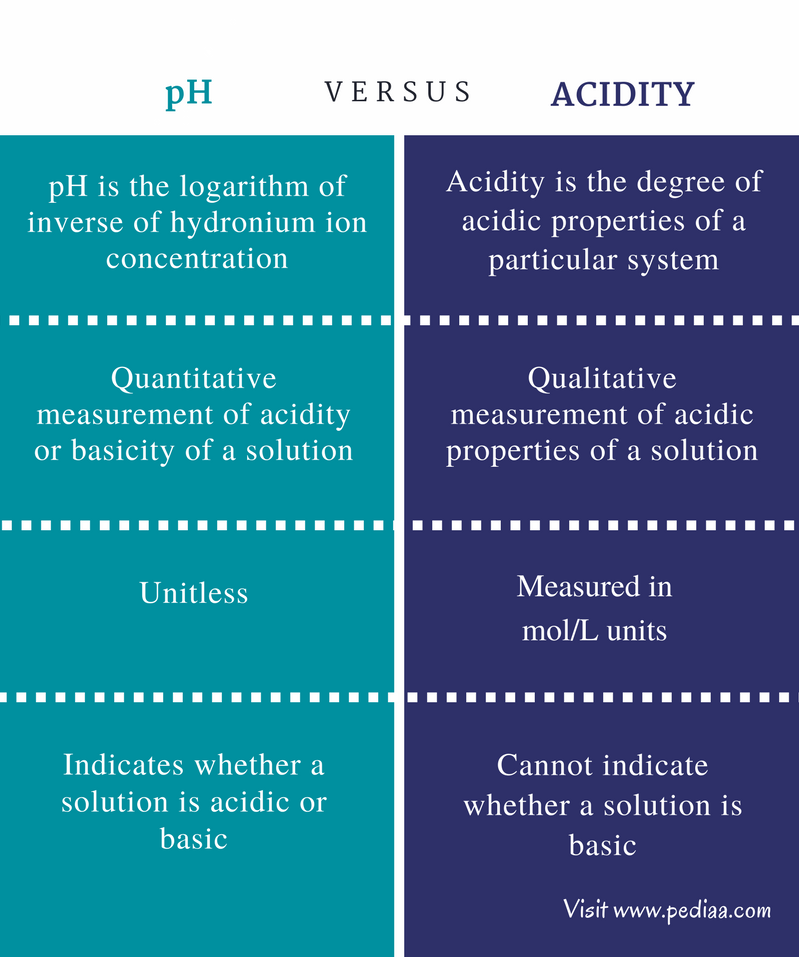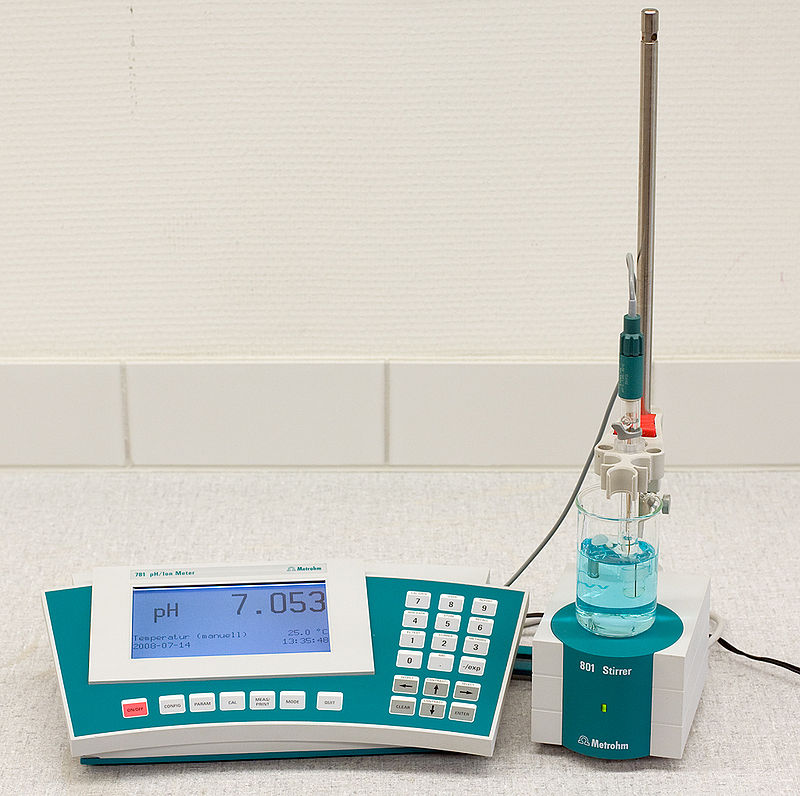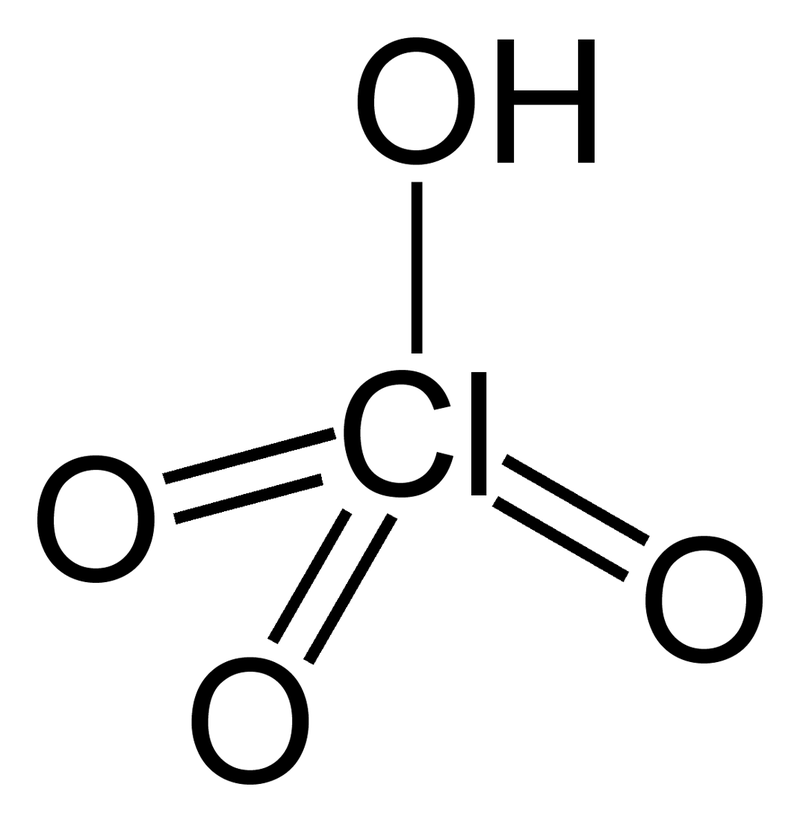# Difference Between pH and Acidity

## Main Difference – pH vs Acidity

pH and acidity are related terms that explain one another. Acidity is the amount of hydronium ions present in a solution. pH is the scale used to measure the amount of hydronium ions present in a solution. Therefore, pH can quantify the acidity of a system. These terms are often used in aquatic chemistry which explains the behavior and properties of water bodies. The main difference between pH and Acidity is that pH is a quantitative measurement of acidity or basicity of a solution whereas acidity is a qualitative measurement of acidic properties of a solution.

### Key Areas Covered

1. What is pH
– Definition, Values, Properties
2. What is Acidity
– Definition, Properties
3. What is the Relationship Between pH and Acidity
– Outline of Common Features
4. What is the Difference Between pH and Acidity
– Comparison of Key Differences

Key Terms: Acid Dissociation Constant, Acidity, Basicity, Hydronium Ion, Litmus, Monoprotic, pH, Strong Acids, Weak Acids## What is pH

pH is the scale that is used to measure the acidity or basicity of a system. Generally, the scale of pH is given from 1 to 14. These pH values have no units since they are logarithmic values. The pH can be defined as the logarithm of the inverse of the hydronium ion concentration. This can be given in mathematical symbols as below.

pH  =    -log10[H3O+(aq)]

The pH of a system can indicate whether the system is acidic or basic. pH 7 is considered as the neutral pH. This is because there is no net acidity or basicity at pH 7. But if a particular system has a pH value below pH 7, then that system is known as an acidic system. Here, more acidic species are present. If the pH of a system is above pH 7, then that system is called a basic system. Here, more basic species are present.

When calculating the pH value of a system, the concentration of hydronium ions is taken in unit mol/L. Therefore, in order to calculate the pH of a system, one should first measure the concentration of H3O+ and then use it in the above equation. When it comes to basicity, the pOH value is measured instead of pH. The pOH is calculated as same as the pH, but the OH concentration is measured there first.Figure 1: pH Meter

The pH can be easily measured using pH meters. One such type of pH meter is shown in the above image. The pH of a solution can be measured by dipping the probe of the pH meter in that solution. After the dipping, button “read” should be pushed. Then the pH of the solution is displayed on the screen.

## What is Acidity

Acidity is the degree of acidic properties of a particular system. The acidity is indicated by the presence of hydronium ions (H3O+) in a solution. Therefore, the Acidity can also be defined as the amount of hydronium ions present in a particular system. The acidity of a solution is measured with pH. Substances with high acidic characteristics are called acids. These substances are able to release protons (H+ ions) through ionization and can form hydronium ions in the solution. These hydronium ions cause the acidity of the solution. According to the ionization process, there are strong acids and weak acids. Strong acids can be ionized completely and release all possible protons to the solution. Weak acids partially ionize and release some of the protons resulting in an equilibrium.

The acid dissociation constant gives an idea about the acidity of a solution. For example, let us consider the dissociation of an acid “HA”.

HA   +   H2O     ↔    A   +    H3O+

The acid dissociation constant of HA is given as below.

Ka = [A][ H3O+] / [HA]

According to the above equation, changes in the hydronium ion concentration can change the Ka value. Therefore, higher the Ka value in a solution, more acidic it is. If the Ka value is lower, the solution has a lower acidity.

Some acids are able to release one proton (or form one hydronium ion) whereas other acids can release more protons. Therefore, the acidity of a solution also depends on how much hydronium ions are formed by a single molecule of the acidic substances present there.

Acidity can be easily determined by using Litmus papers. If blue Litmus is turned to red color when it is dipped in a particular solution, then that solution can be recognized as an acidic solution.Figure 2: Perchloric acid

The above image shows the structure of Perchloric acid. It has one proton (H+ ion) that can be released. So it is a monoprotic acid. The aqueous solutions of perchloric acid are high in acidity.

## Relationship Between pH and Acidity

• Both pH and Acidity can measure the acidic properties of a solution.
• pH is the quantitative measurement of acidity.
• Since acidity is the amount of hydronium ions present in a solution, pH is the logarithmic value of inverse of acidity.
• Higher the pH value is, lower the acidity of a system.
• Lower the pH value, higher the Acidity of a system.

## Difference Between pH and Acidity

### Definition

pH: The pH can be defined as the logarithm of inverse of the hydronium ion concentration.

Acidity: Acidity is the degree of acidic properties of a particular system.

### Nature

pH: pH is a quantitative measurement of acidity or basicity of a solution.

Acidity: Acidity is a qualitative measurement of acidic properties of a solution.

### Units

pH: pH value is unitless.

Acidity: Acidity can be measured in mol/L units.

### Basicity

pH: pH indicates whether a solution is acidic or basic.

Acidity: Acidity cannot indicate whether a solution is basic.

### Conclusion

Pure water is given pH value 7 because it has no acidic or basic species dissolved. But the water we normally use often has a pH value that can range from 6.5 to 7.5. This is because many chemical species are dissolved in water since water is a good solvent. However, the acidity of water is indicated by its pH. The pH values below 7 are known acidic. If the pH value is very low (about pH=2) then those solutions are called strongly acidic and pH values near the pH 7 (but below 7) are called weakly acidic. Therefore, it is very important to understand the difference between pH and acidity and their relationship.

##### References:

1.”Acids, Bases, & the pH Scale.” Science Buddies. N.p., n.d. Web. Available here. 17 July 2017.
2.Hunt, Dr. I. R. “Acidity and Basicity.” Ch 1 : Acidity and Basicity. N.p., n.d. Web. Available here. 17 July 2017.

##### Image Courtesy:

1. “Perchloric-acid-2D” Von Benjah-bmm27 – Eigenes Werk (Gemeinfrei) via Commons Wikimedia
2. “PH Meter” By Datamax – Own work (Public Domain) via Commons Wikimedia